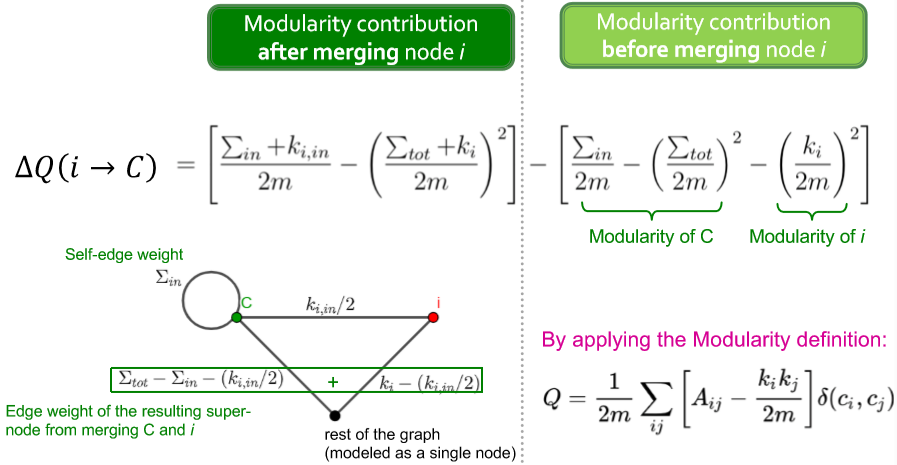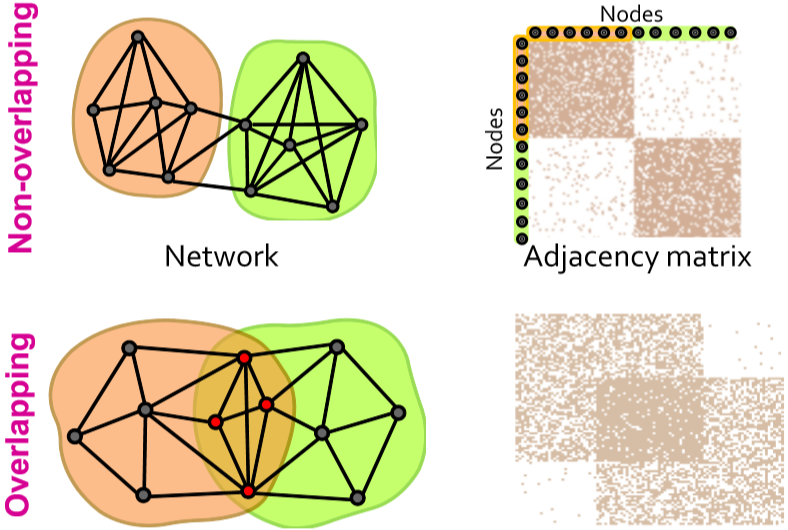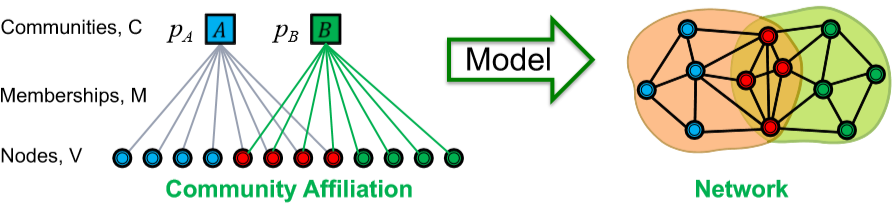# 【图机器学习】cs224w Lecture 4 - 社区结构

## Community

1. 结构上连接紧密的边的社会性更强；跨度大的边连接了网络不同的两个或多个领域反而社会性不稳定
2. 从信息传播的角度来看，跨度大的边能传递不同领域的信息，在找工作方面更有利；而结构上连接密的边过于冗余因此无法提供新的信息

Granovetter 的这个理论在后来电话网络中得到了印证，即连接更强的边一般都有更频繁的电话联络。这里提到一个 edge overlap 需要记录一下，它衡量了两个点间连接的紧密程度。当某条边是 local bridge 时，重叠率为 $0$

$O_{ij}=\frac{|(N(i)\cap N(j))\text{\textbackslash}\{i,j\}|}{|(N(i)\cup N(j))\text{\textbackslash}\{i,j\}|}$

$Q\propto\sum_{s\in S}[(\#\ edges\ within\ group\ s)-(expected \#\ edges\ within\ group\ s)]$

### Configuration Model

\begin{aligned}E_{edge}&=\frac12\sum_{i\in N}\sum_{j\in N}\frac{k_ik_j}{2m} \\ &=\frac12\frac1{2m}\sum_{i\in N}k_i(\sum_{j\in N}k_j) \\ &=m\end{aligned}

\begin{aligned}Q(G,S)&=\frac1{2m}\sum_{s\in S}\sum_{i\in s}\sum_{j\in s}(A_{ij}-\frac{k_ik_j}{2m}) \\ &=\frac1{2m}\sum\limits_{ij}[A_{ij}-\frac{k_ik_j}{2m}]\delta(c_i,c_j)\end{aligned}

### Louvain Algorithm

• 速度快
• 收敛快
• 结果好
• 支持嵌套社区结构

1. 在局部范围内交换节点的社区，如果交换后能使 modularity 增大则保留交换，否则回退
2. 在第一步收敛后将所有属于同一社区的节点汇聚为一个超级节点，然后根据原图结构连接这些超级节点形成新的图，而边的权重是所有对应边的权重之和

$\Delta Q(i\rightarrow C)=\big[\frac{\sum_{in}+k_{i,in}}{2m}-\big(\frac{\sum_{tot}+k_i}{2m}\big)^2\big]-\big[\frac{\sum_{in}}{2m}-\big(\frac{\sum_{tot}}{2m}\big)^2-\big(\frac{k_i}{2m}\big)^2\big]$

• $\sum_{in}$ 社区 $C$ 内边的权重之和
• $\sum_{tot}$ 与社区 $C$ 内所有点相连的边的权重
• $k_{i,in}$ 节点 $i$ 和社区 $C$ 内所有点的连接的权重和
• $k_i$ 与节点 $i$ 连接的所有边的权重和

(目前先理解加入这一步，但没有自己推导；而移除的表达式没有推，后面有时间会补上)## BigCLAM

Louvain 虽然好处多多应用也广，但有个缺陷，它只能给每个节点分配一个社区。然而现在社会结构复杂，一个人可能参加多个社团或属于多个社区，这样就是 overlap 的问题。用邻接矩阵来表示更直观$l(F)=\sum\limits_{(\mu,\nu)\in E}\log(1-\exp(-F_{\mu}F_{\nu}^T))-\sum\limits_{(\mu,\nu)\notin E}F_{\mu}F_{\nu}^T$

1. 随机初始化 AGM 的参数 $F$
2. 固定其他节点的社区成员关系，更新节点 $\mu$

$\nabla l(F_{\mu})=\sum\limits_{\nu\in\mathcal{N}(\mu)}F_{\nu}\frac{\exp(-F_{\mu}F_{\nu}^T)}{1-\exp(-F_{\mu}F_{\nu}^T)}-\sum\limits_{\nu\notin\mathcal{N}(\mu)}F_{\nu}$

1. 这里 $i$$C$ 的权重为什么是 $k_{i,in}/2$，不应该就是 $k_{i,in}$ 吗？ ↩︎

posted @ 2020-04-05 16:14  WineChocolate  阅读(461)  评论(0编辑  收藏